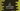# C++ program to print the prime factors of a number## C++ program to print the prime factors of a number:

The factors of a number are numbers, which can divide the number exactly. For example, for the number 15, we have four factors: 1, 3, 5 and 15.

A factor is called a prime factor if it is a prime number. A number is called a prime number, if it is divisible by 1 and itself.

We can use a loop to print all prime factors of a number. Note that 1 is not a prime number.

## Method 1: By using a for loop:

Let’s try to write this by using a for loop:

``````#include <iostream>
using namespace std;

bool isPrime(int n)
{
for (int i = 2; i <= n / 2; i++)
{
if (n % i == 0)
{
return false;
}
}
return true;
}

int main()
{
int num;

cout << "Enter a number: " << endl;
cin >> num;

for (int i = 2; i <= num; i++)
{
if (num % i == 0)
{
if (isPrime(i))
{
cout << i << " is a prime factor of " << num << endl;
}
}
}
}``````

Here,

• isPrime is a method to check if a number is prime or not. It takes one integer value as the parameter and it returns one boolean value. It returns true if the number is prime, else it returns false.
• The for loop runs from 2 to number/2 and if it finds any value that can divide number perfectly, it returns false.
• If it don’t find any value that can divide the number perfectly, it returns true, i.e. it is a prime number.
• Inside main, it asks the user to enter a number and stores that value in num.
• The for loop runs from i = 2 to number that user has entered. If it finds that i can divide the number perfectly, it checks if i is a prime or not. It prints one message if it is prime.

### Sample output:

If you run this program, it will give output as like below:

``````Enter a number:
100
2 is a prime factor of 100
5 is a prime factor of 100

Enter a number:
211
211 is a prime factor of 211

Enter a number:
2013
3 is a prime factor of 2013
11 is a prime factor of 2013
61 is a prime factor of 2013``````

## Method 2: By using a while loop:

We can also use a while loop in a similar way. Below is the complete program:

``````#include <iostream>
using namespace std;

bool isPrime(int n)
{
int i = 2;
while (i <= n / 2)
{
if (n % i == 0)
{
return false;
}
i++;
}
return true;
}

int main()
{
int num, i = 2;

cout << "Enter a number: " << endl;
cin >> num;

while (i <= num)
{
if (num % i == 0)
{
if (isPrime(i))
{
cout << i << " is a prime factor of " << num << endl;
}
}
i++;
}
}``````

If you run it, it will give similar output.

## Method 3: Recursive way:

A method is called a recursive method if it calls itself in a repeated manner. We can change the isPrime method to a recursive method to find out if a number is prime or not.

``````#include <iostream>
using namespace std;

bool isPrime(int n, int i)
{
if (i > n / 2)
{
return true;
}

if (n % i == 0)
{
return false;
}

return isPrime(n, i + 1);
}

int main()
{
int num, i = 2;

cout << "Enter a number: " << endl;
cin >> num;

while (i <= num)
{
if (num % i == 0)
{
if (isPrime(i, 2))
{
cout << i << " is a prime factor of " << num << endl;
}
}
i++;
}
}``````

Here,

• Only isPrime is changed
• It also takes the i value as the second argument. The first argument is the number itself.
• isPrime is a recursive method. It calls itself again and again to find out if the provided number is prime or not.
• If you look closely, you can see that it is similar to the non-recursive one. If it finds that i is more than n/2, it returns true. If n is perfectly divisible by i, it returns false, else it calls itself recursively by incrementing the value of i to get the final result.

If you run this program, it will print similar output.